Function Repository Resource:

# CobwebPlot

Visualize one-dimensional iterated functions

Contributed by: Paul Abbott
 ResourceFunction["CobwebPlot"][f,x0,n,{a,b}] plots the real function f along with the diagonal line over the range a to b and, starting from x0, computes n iterates of the function, reflecting each iteration of the function in the diagonal line.

## Details and Options

ResourceFunction["CobwebPlot"] has the same options as Graphics.
ResourceFunction["CobwebPlot"] is useful for visualizing the logistic map and other functions.

## Examples

### Basic Examples (1)

Display a cobweb plot of 10 iterations of the map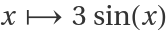starting at x0=2:

 In:=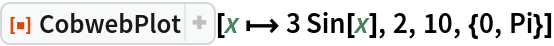Out=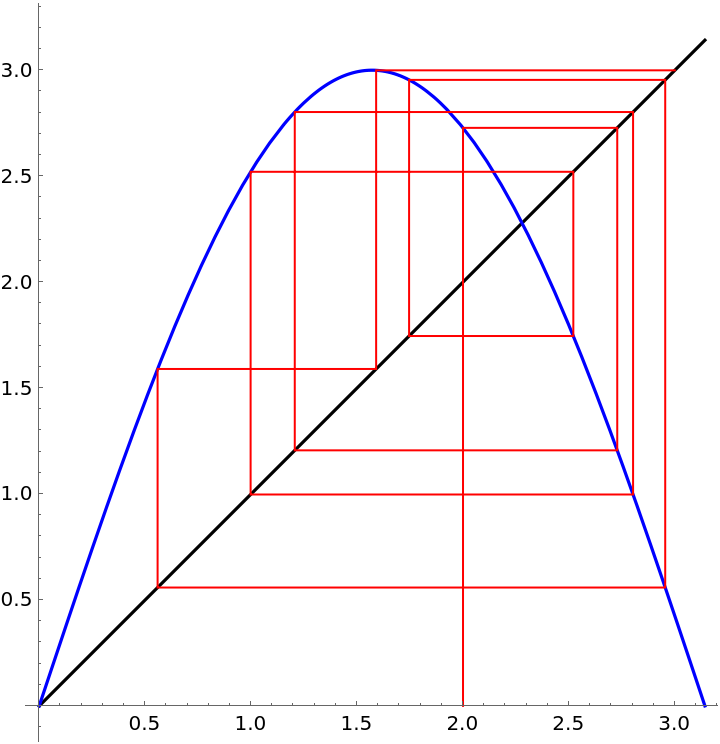### Options (1)

Show the cobweb as a dashed red line:

 In:=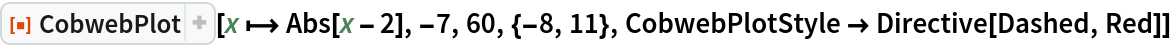Out=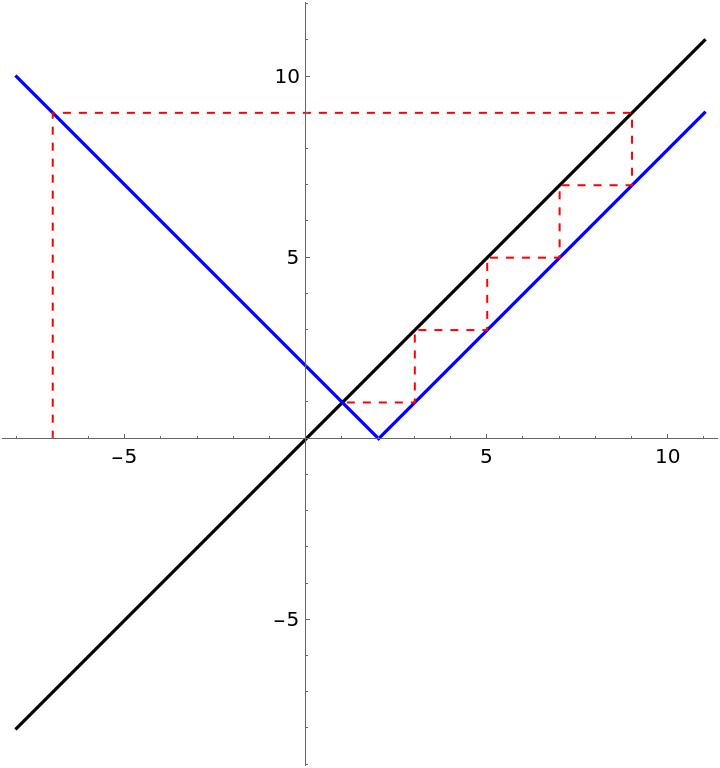### Applications (1)

For the logistic map, xn+1:=α xn(1-xn), display the cobweb plot of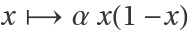, varying the parameter α, the starting value x0 and the number of iterations n:

 In:=Out=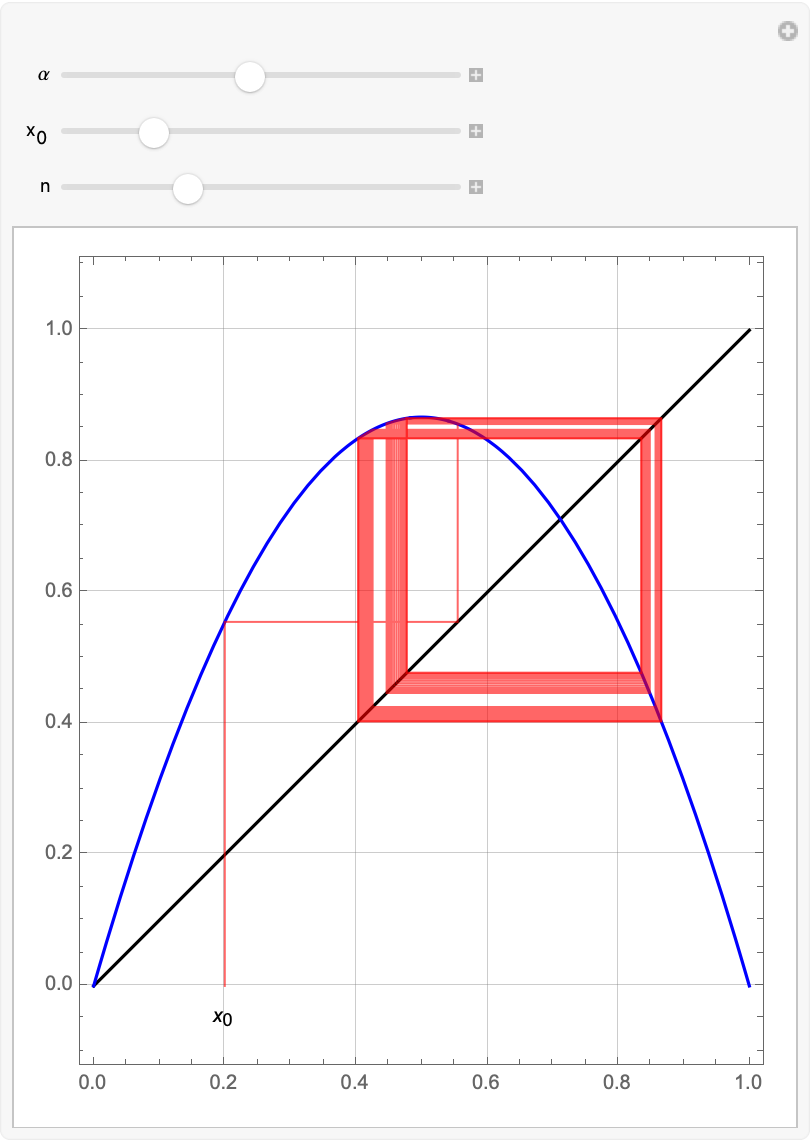### Properties and Relations (1)

Display the logistic map along with the associated Feigenbaum diagram:

 In:=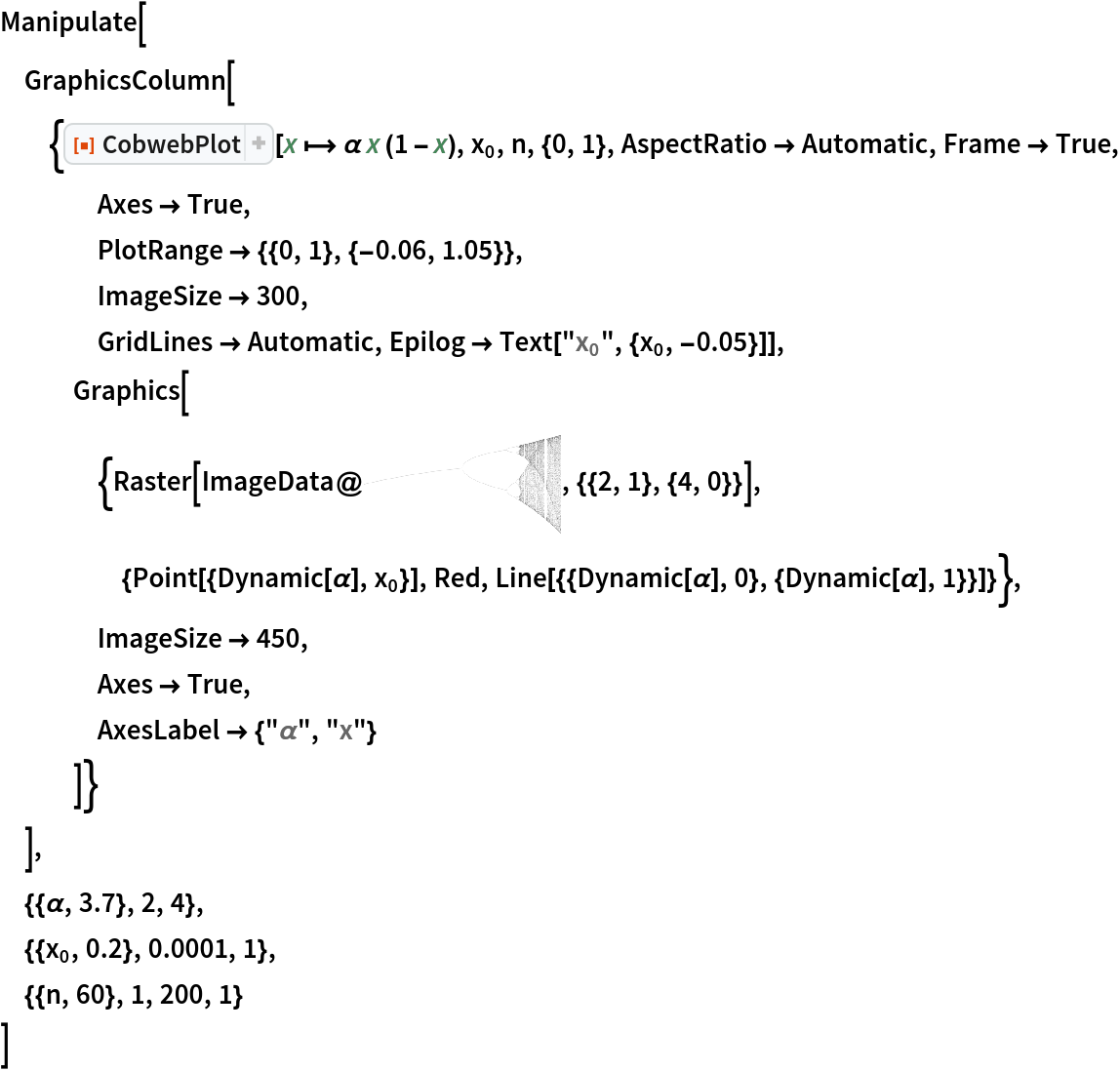Out=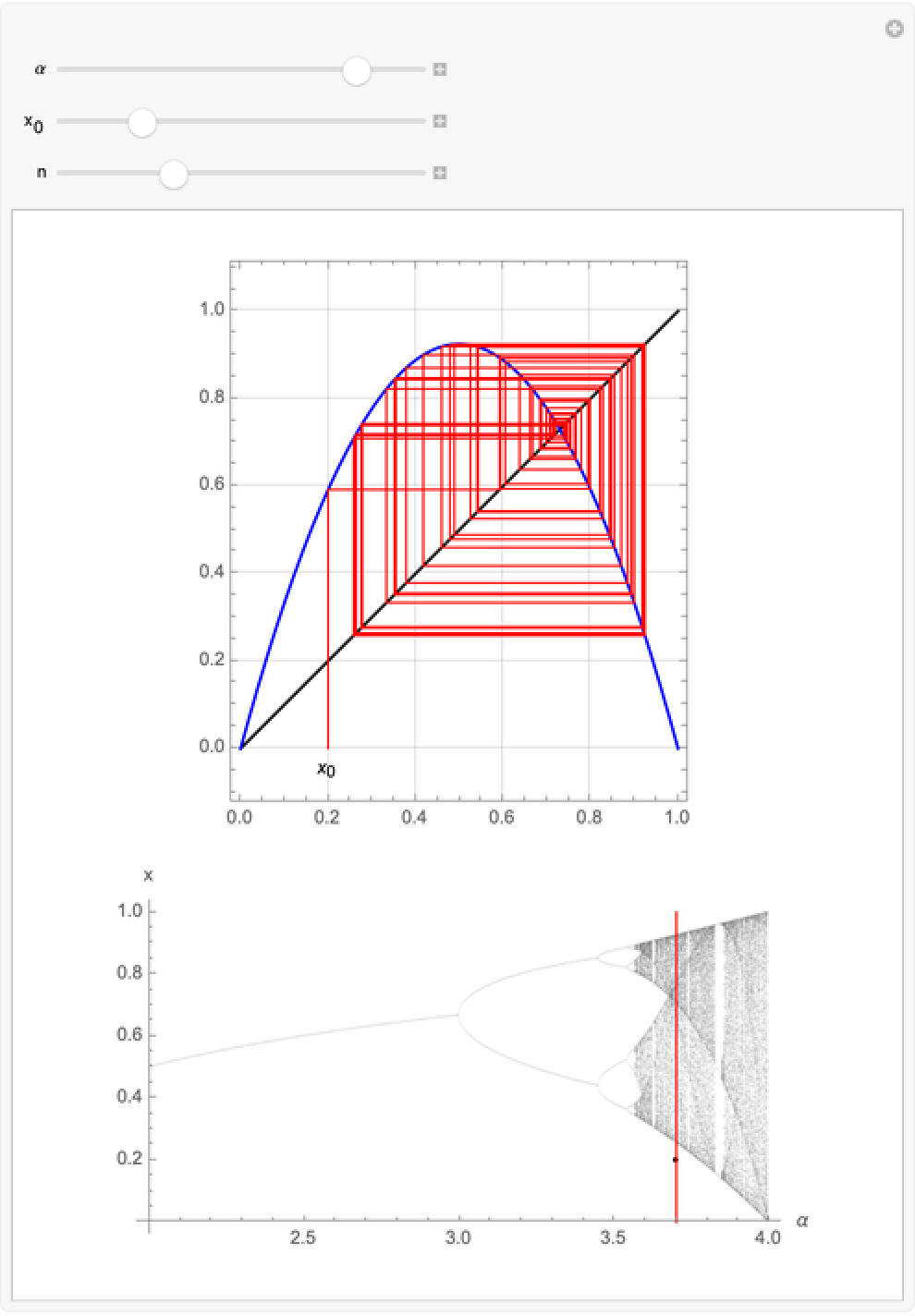### Neat Examples (1)

For integer values the map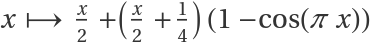corresponds to the (reduced) Collatz (or 3X+1) function: if x is even, return x/2, but if x is odd, return (3x+1)/2. For small starting integers n, the Collatz map iterates to the cycle 1↔2 and the cobweb plot converges to the yellow square:

 In:=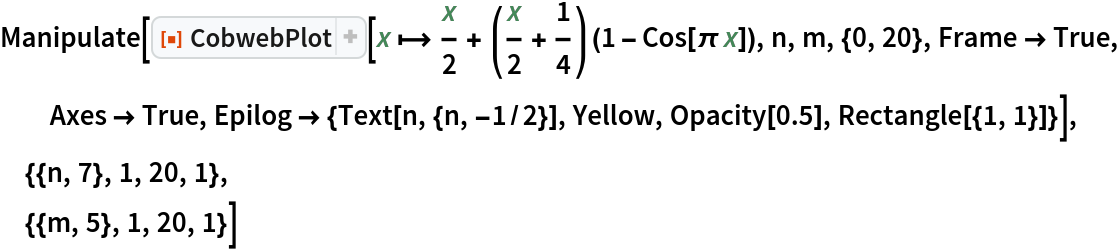Out=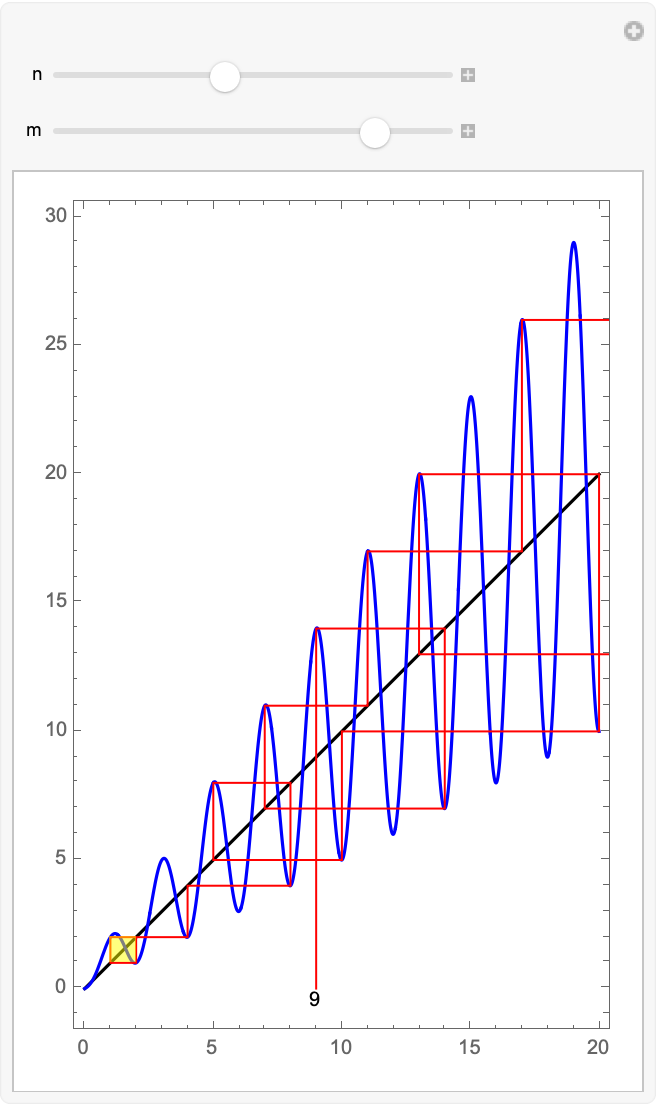Paul Abbott

## Version History

• 1.0.0 – 08 February 2021